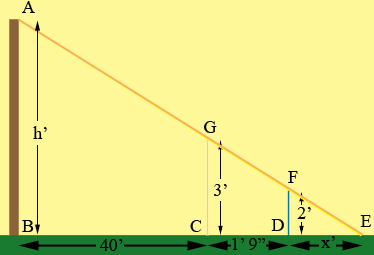SEARCH HOMEMath Central Quandaries & QueriesQuestion from Wanda, a parent: One boy holds a yardstick vertically at a point 40 feet from the base of the flagpole. The other boy backs away from the pole to a point where he sights the top of the pole over the top of the yardstick. If his position is 1 ft 9 in from the yardstick and his eye level is 2 ft above the ground, find the height of the flagpole.Hi Wanda,

Did you draw a diagram?The second boy's eye, the top of the yard stick and the top of the flagpole form a straight line. I extended this line to meet the ground at E and called the distance the second boy to the ground |DE| = x feet.

The triangles GCE and FDE are similar and hence

|CE|/|GC| = |DE|/|FD|
(1' 9" + x')/3' = x'/2'

But 1' 9" = 1.75' and hence you can solve the above equation for x.

Now use the fact that triangles ABE and FDE are similar to find h.

PennyMath Central is supported by the University of Regina and The Pacific Institute for the Mathematical Sciences.